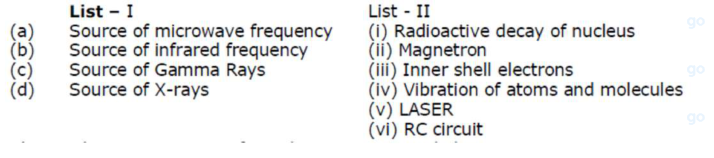# Match List - I with List - II. List - I List - IIQuestion:

Match List - I with List - II. List - I List - IIChoose the correct answer from the options given below :

1. (1) $(a)-(i i),(b)-(i v),(c)-(i),(d)-(i i i)$

2. (2) (a) - (vi), (b) - (iv), (c) - (i), (d) - (v)

3. (3) (a) - (ii), (b) - (iv), (c) - (vi), (d) - (iii)

4. (4) $(\mathrm{a})-(\mathrm{vi}),(\mathrm{b})-(\mathrm{v}),(\mathrm{c})-(\mathrm{i}),(\mathrm{d})-(\mathrm{i} v)$

Correct Option: 1

Solution:

(1)

(a) Source of microwave frequency - (ii) Magnetron

(b) Source of infra red frequency - (iv) Vibration of atom and molecules

(c) Source of gamma ray - (i) Radio active decay of nucleus

(d) Source of X-ray - (iii) inner shell electron